# Quiz 5: Adjustable and Floating Rate Mortgage Loans

Price level adjusted mortgage (PLAM) is a kind of mortgage where the mortgage payments get adjusted for inflation. The new mortgage payments after the adjustments are computed using the adjusted balance. In this kind of mortgages interest rate does not get varied while the principal changes. Usually the changes are reflected by index-CPI. For implementation of these changes the intervals are agreed upon by lender and borrower at frequent intervals. As PLAM is a kind of remedy used to imbalance the problems for the savings institutions. In order to help reduce the risks associated with uncertainties of inflation and its impact over interest rates, it is suggestive for the lenders to originate mortgages at interest rates that which reflect the expectations of real interest rate in addition to a risk premium. This is needed to avoid the likelihood of loss that occurs due to getting defaulted upon a mortgage taken. The mortgage amount is \$95,000; initial interest rate is 4%, compounded monthly; the points are 6%; period is 30 years and inflation rate is 6% for next five years. (a) Calculate the payments at the beginning of each month for five years are as follows: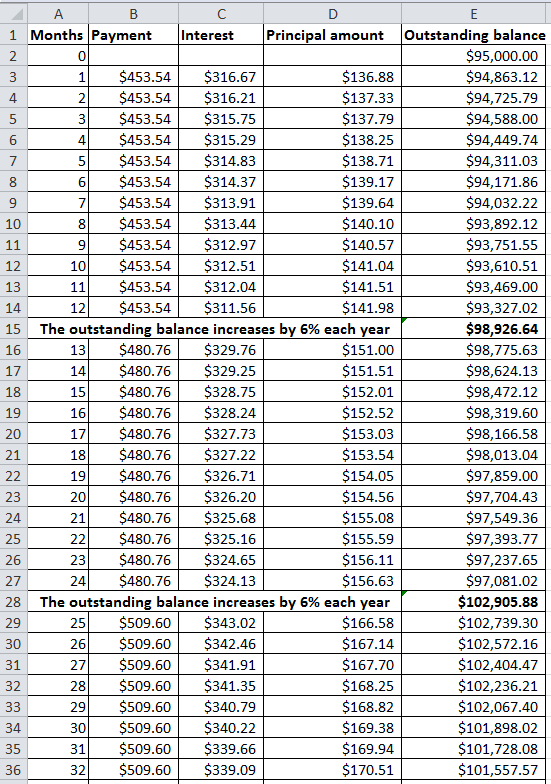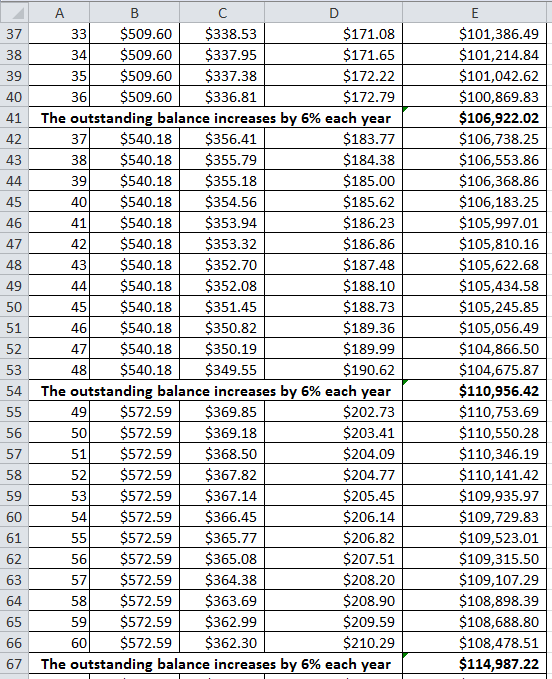Formulas for above values are as follows: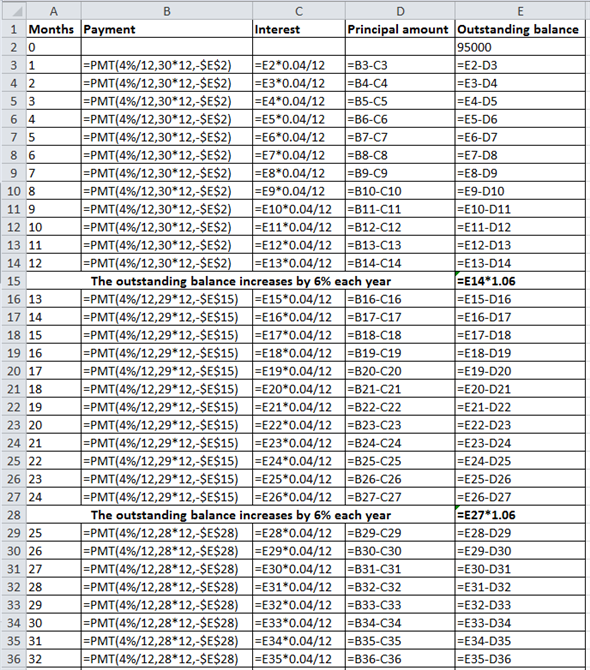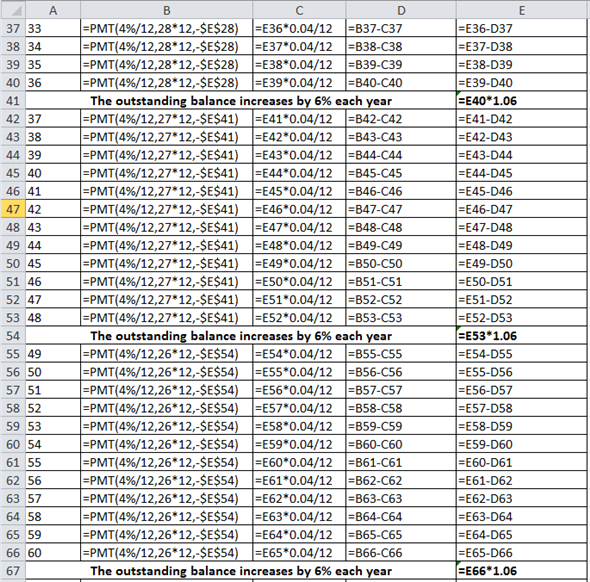The following table shows the yearly payments as follows: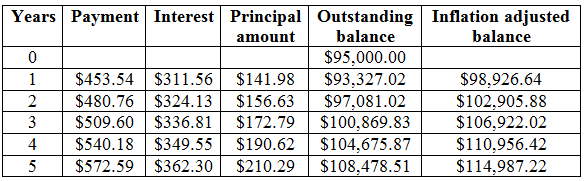(b) From the above calculation, it can be stated that the loan balance at the end of fifth year is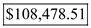. (c) Calculate the yield to the lender on the mortgage using MS-EXCEL "IRR" Function as follows: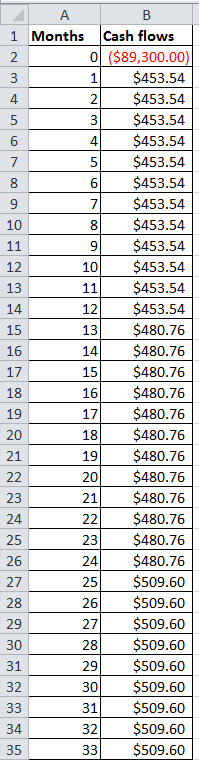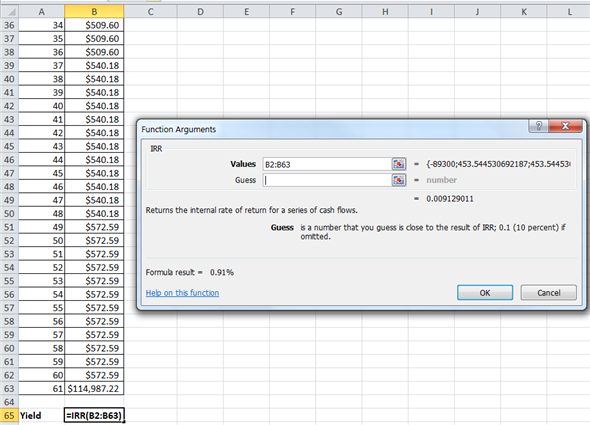Explanation: IRR can be calculated using Excel "IRR" function. The Excel steps to calculate IRR function are as follows: • First go to the Menu bar of Excel and select 'Formulas' option • Select Insert Function'(fx) • Then select category as Financial • Then select "IRR" and click OK • Then the Function Argument window will open. Now, input the given data in the required field • Click OK • The formula will display the final answer as 0.91% Calculate the effective annual yield on mortgage as follows:Therefore, the effective annual yield on mortgage is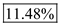. Working Notes: • Cash flow at 0 th month is -\$89,300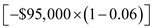. • Cash flow at 61 st month is the outstanding balance of \$114,987.22 as calculated in sub-part (a).
Introduction: Fixed interest rate payment on mortgage loan is the system of interest payments on the mortgage loan with fixed annual interest rate. The rate of interest on loan is established by the willingness of the borrowers to pay the interest. Problems with fixed interest rate of interest: The fixed interest payments are Constant Amortisation Mortgage loan (CAM) and Reverse Annuity Mortgage (RAM). These evolutions could not address the problems of lenders due to the following reasons: • The fixed interest rate does not consider the change in inflation and inflationary expectations. • The risk premium is not covered for the borrower of mortgage loan by fixed rate of interest. • The market rate of interest is not considered under fixed rate of interest on mortgage loan. Overcoming the problems of fixed interest rate: The two methods for computation of flexible rate of interest are adopted to overcome the problems of fixed interest rate: Price Level Adjusted Mortgage (PLAM): The uncertainty of interest rate due to the effect of inflation is addressed by the Price Level Adjusted Mortgage (PLAM) technique. The interest rate under PLAM method is computed in the following manner: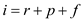Here, i = Interest rate r = Real rate of interest p = Risk premium f = Expected inflation Therefore, the computation of interest rate under PLAM considers the expected inflation. Adjustable Rate Mortgage: The adjustable rate mortgage is another technique to measure the interest rate on mortgage loans. The main terms used under ARM method are as follows: 1. Index 2. Margin 3. Composite rate 4. Expected start rate 5. Actual start rate 6. Teaser rate 7. Reset date 8. Negative amortization 9. Caps 10. Floors 11. Assumability 12. Discount points 13. Prepayment privilege 14. Conversion option